### Total 18 Videos found in Category "California Standards Test: Geometry"

Title
 1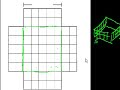CA Geometry: Area, Circumference, Volume 2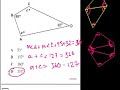CA Geometry: Area, Pythagorean Theorem 3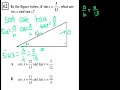CA Geometry: Basic Trigonometry 4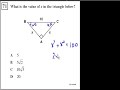CA Geometry: Circle Area Chords Tangent 5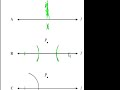CA Geometry: Compass Construction 6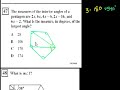CA Geometry: Deducing Angle Measures 7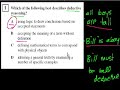CA Geometry: deductive reasoning 8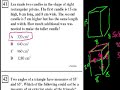CA Geometry: Exterior Angles 9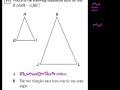CA Geometry: More on congruent and similar triangles 10CA Geometry: More Proofs 11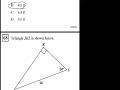CA Geometry: More Trig 12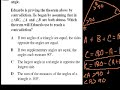CA Geometry: Proof by Contradiction 13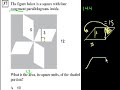CA Geometry: Pythagorean Theorem, Area 14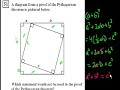CA Geometry: Pythagorean Theorem, Compass Constructions 15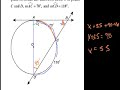CA Geometry: Secants and Translations 16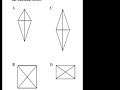CA Geometry: Similar Triangles 1 17CA Geometry: Similar Triangles 2 18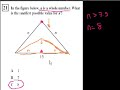CA Geometry: Triangles and Parallelograms

Say and share some thing about these videos...